# Kerala SSLC Chemistry Model Question Paper 2 English Medium

Students can Download Kerala SSLC Chemistry Model Question Paper 2 English Medium Pdf, Kerala SSLC Chemistry Model Question Papers helps you to revise the complete Kerala State Board New Syllabus and score more marks in your examinations.

## Kerala SSLC Chemistry Model Question Paper 2 English Medium

General Instructions:

1. The first 15 minutes is the cool off time. You may use the time to read and plan your answers.
2. Answer the questions only after reading the instructions and questions thoroughly.
3. Questions with marks series 1, 2, 3 and 4 are categorized as sections A, B, C and D respectively.
4. Five questions are given in each section. Answer any four from each section.
5. Answer each question by keeping the time.

Time: 1½ Hours
Total Score: 40 Marks

Section  – A

(Answer any 4 questions from 1 to 5. Each question carries 1 score) (4 × 1 = 4)

Question 1.
Which one of the following is not possible?
a) 2s 2
b) 3p3
c) 2d5
d) 4F2
NH4OHQuestion 2.
Which metal cannot displace copper from CuSO4. solution.
a) Magnessium
b) Silver
c) Zinc
d) Iron
Silver

Question 3.
The substance obtained at the cathode when NaCl solution is electrolysed is …………..
a) Sodium
b) Chlorine
c) Hydrogen
d) Sodium hydroxide
Hydrogen

Question 4.
The chemical formula of liquor ammonia is ………..
a) NH4OH
b) NH4Cl
c) (NH2)SO4
d) NH3
NH4OH

Question 5.
The symbol of carboxylase functional group is ……………
a) -OH
b) – COOH
c) -CH3
d) – O –
-COOHSection – B

(Answer any 4 questions from 6 to 10. Each question carries 2 scores)(4 × 2 = 8)

Question 6.
The subshell electron Configuration of certain elements are given below.
Find out the wrong ones and correct them (symbols are not real)
a) 12P = 1s22s22p63s2
b) 6Q = 1s22s23s2
c) 178R = 1s22s22p63s23p6
d) 20S = 1s22s22p63s23p63d2
Q – 1s22s22p2
S – 1s22s22p63s23p63d64s2

Question 7.
Calculate the number of molecules in 90g of water.
(Hint -Atomic mass H = 1 O = 16)
1 GMM of water = 18 g.
90 g water = $$\frac{90}{18}$$ = 5 mol
No. of molecules in 5 mol = 5 x 6.022 x 1023Question 8.
Observe the solutions and the metals placed in three test tubes.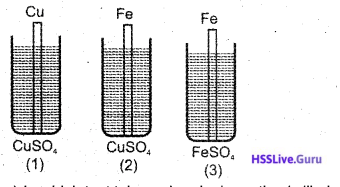a) In which test tube a chemical reaction is likely to take place? Why. (1)
b) Write the name of the reaction. (1)
c) Write the equation of the redox reaction taking place here. (1)
a) In test tube 2. More reactive iron displaces copper from CuSO4 solution.
b) Displacement reactions
c) Fe + Cu2+ → Fe2+ + Cu

Question 9.
Metallurgy involves all the process leading to the separation of a pure metal from its ore.
a) Distinguish a mineral from an ore. (1)
b) What type of ores are usually concentrated by froth floatation process. Given an example. (2)
a) Elements or their compounds, occurring naturally and obtained by mining are called minerals, Ore is the mineral from which a metal is extracted economically, easily and quickly.
b) Sulphide ores eg. Copper pyrites.Question 10.
Analyse the chemical equations given below and answer the questions that follow.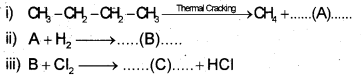a) Identify A, B and C. (2)
b) Write what type of chemical reactions (i) and (ii) are (1)
a) A = CH3-CH = CH2 or C3H6
B = CH3 – CH2 – CH3 or C3H8
C = CH3 – CH2 – CH2 – Cl

ii) Substitution reaction

Section – C

(Answer any 4 questions from 11 to 15. Each question carries 3 scores) (4 × 3 = 12)

Question 11.
The outermost electronic configuration of an element , X is 3s2 3p1. Answer the questions given below related
to this element. (Symbol of the element is not actual).
a) What is the atomic number of this element? (1)
b) Write the group number and period number of X? (1)
c) Write the formula of the oxide of this element?(1)
a) 13
b) Group-13, Period-3
c) X2O3Question 12.
The atomic masses of certain elements are S = 32, O = 16, H = 1,C = 12
a) Find out 1 GMM of H2SO4 (1)
b) Find out the number of molecules in 220 gCO2(2)
c) What will be the volume of 1 mole SO2 at STP?(1)
a) 98g
b) 220g CO2 = $$\frac{220}{44}$$ = 5 mol
No. of molecules in 5 mol = 5 x 6.022 x 1023
c) 22.4 L

Question 13.
Analyse the picture of an electro chemical cell given below, and answer the questions that follow.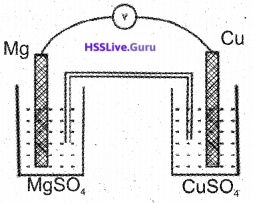a) Which are the anode and cathode in this cell?(1)
b) Write the equation of chemical reaction taking
place at both of the electrodes. (2)
c) Write the equation of the redox reaction.
a) Anode – Mg
Cathode – Cu
b) At anode Mg →Mg2+ + 2e
At cathode Ca2+ + 2e → Cu
c) Mg + Cu2+ → Mg2+ + CuQuestion 14.
Certain metals their characteristics and the method of refining are tabled below. Match them suitably.(3)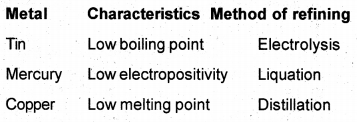Tin-Low melting point – Liquation
Mercury – Low boiling point – Distillation
Copper – Low electropositivity – Electrolysis

Question 15.
The structural formula of an organic compound is given below.
CH3-CH2-CH2-CH2-OH
a) Write the IUPAC name of the compound. (1)
b) Write the structure of a position isomer of this compound. (1)
c) Write the structure of a functional group isomer of this compound. (1)
a) Butan -1 – ol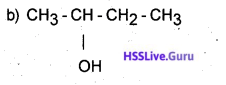(c) CH3 – CH2 – O – CH2 – CH3 (OR)
CH3 – O – CH2 – CH2 – CH3Section – D

(Answer any 4 questions from 16 to 20. Each question carries 4 scores)(4 × 4 = 16)

Question 16.
The chemical equation showing the preparation of 8 -10% of ethnol is given belowa) Write the products A and B. (1)
b) How the product obtained is converted in to rectified spirit? (1)
c) What is denatured spirit? (1)
a) A = C6H12O6
A = CO2
b) By fractional distillation
c) Ethanol added with poisonous substances to avoid its misuse as a beverage.

Question 17.
Analyse the compounds given belowa) Which among these compounds is an ester?(1)
b) Choose the compounds required to form this ester and write the chemical equation. (1)
c) Write any two uses of esters. (1)
a) CH3-COO-CH3
b) CH3-COOH-CH3-OH →CH3-COO-CH3
c) Used as a flavouring agent for food. Used for making perfumes.

Question 18.
Two reversible reactions are given below:
i) H2(g) + l2(g)⇌ 2Hl(g)
ii) N2(g) + 3H2(g) ⇌ 2NH3(g) + Heat
a) In which reaction change of pressure has no effect.
b) Suggest two methods to increase the amount of product in the second reaction.
c) Even though the forward reaction is exothermic the temperature is maintained at 450°C. Why?
a) Pressure has no effect in reaction -1. Because there is no change in number of molecules due to forward and backward reactions.
b) Increase pressure Increase the concentration of the reactants Remove the products continuously from the system.
c) 450°C is the optimum temperature of this reaction. At this temperature maximum product is obtained. At low temperature NH3 decomposes back in the reactants.Question 19.
At one end of a glass tube cotton soaked in cone.
HCl and at the other end of it cotton soaked in cone.
NH4OH solution is inserted.a) What will be the observation after sometime?
b) What will happen if the glass tube is heated?
c) Write equations of the chemical reactions in a and b
a) A white precipitate is formed inside the glass tube. NH3 gas and HCI gas combines to form NH4Cl.
b) The precipitate disappears on heating. On heating NH4Cl decomposes into NH3 and HCl

c) NH3 + HCI → NH4Cl
NH4Cl → NH3 + HCl

Question 20.
Match those given in columns A, B and C suitably.

 A B C Bayles Law Reversible Reaction V&T Charles Law Volume & number of molecules V ∝ 1/p Avagadros Law Volume & pressure V ∝ η Le-Chatelers principle Volume and temperature Equillibrium state